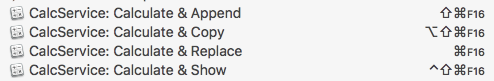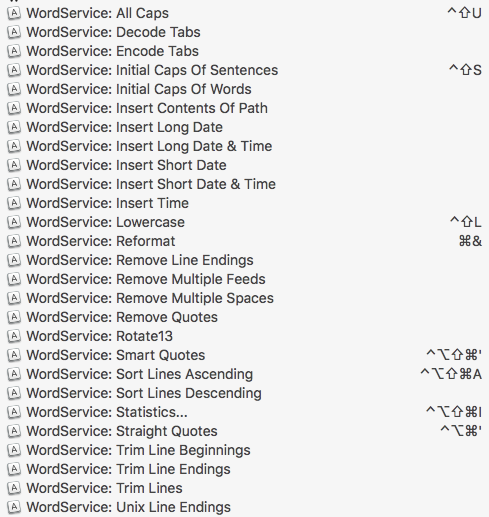# Macro to Sum All Copied Amounts

At the end of the year I want to open all the PDFs with invoices that I've created and quickly copy the invoice amounts and sum them.

I was thinking of a macro that:

• Once started, places all copied amounts in a list
• Displays this list in a little window (e.g. in the upper right corner)
• Sums the amounts copied (added) every time a new amount is added and rewrites the window
• Places the whole list on the clipboard end ends the macro, once the window is closed

Is there someone who has already created such a macro?

there's some macros to sum amounts:

I'd say you can pair it with this one to go trough every line:

1 Like

I might begin by splitting this (at least for the moment) into two separate problems:

1. gathering a list, by successive copies, of decimal or currency string lines
2. showing the list of numbers in the clipboard, with a calculated total.

Shown here with Anglo-Saxon number formatting, but should also work with comma decimals:

For part one (gathering a list by copying ) perhaps you could use the Activate Clipboard History action which allows you to:

• see a list of recently copied values
• select and merge some or all of them into a single clipboard (list of lines) with ⌘C

For part two, I would personally reach for an Execute JavaScript action, and the key issue would be to go beyond the built-in parseFloat function (which is parochial – locale ignorant – and only works with Anglo-Saxon decimal points).

Here is a draft which aims for Locale-aware parsing of numbers and currency values, using the ObjC `\$.NSNumberFormatter` interface.

Once you have used the KM Clipboard History to merge a series of numeric lines into the current clipboard, you can try running the following macro to calculate and display the list and total:

JS Source
``````(() => {
'use strict';

ObjC.import('AppKit');

// Rob Trew @2020

// First draft of summing numeric string lines
// (locale-specific floating point or currency values)
// found in the clipboard.

// Ver 0.2

// main :: IO ()
const main = () =>
either(
)(
table => table
)(
bindLR(
clipTextLR()
)(clip => {
const
numberStrings = lines(clip)
.flatMap(
x => isNaN(x) ? (
[]
) : [x.trim()]
),
strTotal = numberStrings.reduce(
(a, x) => a + parseLocaleNum(x),
0
).toLocaleString(),
w = (
maximum(
[strTotal].concat(numberStrings)
.map(length)
)
);

return 0 < numberStrings.length ? (
Right(
'Total:\n\n' + unlines(
numberStrings.map(justifyRight(w)(' '))
) + '\n' + (
'-'.repeat(w) + ' +\n'
) + justifyRight(w)(' ')(strTotal)
)
) : Left('No numbers found in clipboard.');
})
);

// ----------------------- JXA -----------------------

// alert :: String => String -> IO String
s => {
const sa = Object.assign(
Application('System Events'), {
});
return (
sa.activate(),
sa.displayDialog(s, {
withTitle: title,
buttons: ['OK'],
defaultButton: 'OK'
}),
s
);
};

// clipTextLR :: () -> Either String String
const clipTextLR = () => (
v => Boolean(v) && 0 < v.length ? (
Right(v)
) : Left('No utf8-plain-text found in clipboard.')
)(
ObjC.unwrap(
\$.NSPasteboard.generalPasteboard
.stringForType(\$.NSPasteboardTypeString)
)
);

// ---- PARSING LOCALE FLOATS OR CURRENCY VALUES -----

// parseLocaleCurrency :: String -> Float
const parseLocaleCurrency = s =>
// ObjC.import('AppKit')
parseLocaleNumeric(
\$.NSNumberFormatterCurrencyAccountingStyle
)(s);

// parseLocaleFloat :: String -> Float
const parseLocaleFloat = s =>
// ObjC.import('AppKit')
parseLocaleNumeric(
\$.NSNumberFormatterDecimalStyle
)(s);

// parseLocaleNum :: String -> (Num | NaN)
const parseLocaleNum = s =>
parseLocaleFloat(s) || parseLocaleCurrency(s);

// parseLocaleNumeric :: NSNumberFormatterStyle ->
// String -> Either Num NaN
const parseLocaleNumeric = nsNumStyle =>
// ObjC.import('AppKit')
// [NSNumberFormatterCurrencyStyle](
//  https://developer.apple.com/documentation/
//  foundation/nsnumberformatterstyle/nsnumberformattercurrencystyle
// )
s => {
const formatter = \$.NSNumberFormatter.alloc.init;
return (
formatter.locale = \$.NSLocale.currentLocale,
formatter.numberStyle = nsNumStyle,
formatter.numberFromString(s).doubleValue
);
};

// --------------------- GENERIC ---------------------

// Left :: a -> Either a b
const Left = x => ({
type: 'Either',
Left: x
});

// Right :: b -> Either a b
const Right = x => ({
type: 'Either',
Right: x
});

// bindLR (>>=) :: Either a ->
// (a -> Either b) -> Either b
const bindLR = m =>
mf => undefined !== m.Left ? (
m
) : mf(m.Right);

// either :: (a -> c) -> (b -> c) -> Either a b -> c
const either = fl =>
// Application of the function fl to the
// contents of any Left value in e, or
// the application of fr to its Right value.
fr => e => 'Either' === e.type ? (
undefined !== e.Left ? (
fl(e.Left)
) : fr(e.Right)
) : undefined;

// justifyRight :: Int -> Char -> String -> String
const justifyRight = n =>
// The string s, preceded by enough padding (with
// the character c) to reach the string length n.
c => s => n > s.length ? (
) : s;

// length :: [a] -> Int
const length = xs =>
// Returns Infinity over objects without finite
// length. This enables zip and zipWith to choose
// the shorter argument when one is non-finite,
// like cycle, repeat etc
'GeneratorFunction' !== xs.constructor
.constructor.name ? (
xs.length
) : Infinity;

// lines :: String -> [String]
const lines = s =>
// A list of strings derived from a single
// string delimited by newline and or CR.
0 < s.length ? (
s.split(/[\r\n]+/)
) : [];

// list :: StringOrArrayLike b => b -> [a]
const list = xs =>
// xs itself, if it is an Array,
// or an Array derived from xs.
Array.isArray(xs) ? (
xs
) : Array.from(xs || []);

// maximum :: Ord a => [a] -> a
const maximum = xs => (
// The largest value in a non-empty list.
ys => 0 < ys.length ? (
ys.slice(1).reduce(
(a, y) => y > a ? (
y
) : a, ys
)
) : undefined
)(list(xs));

// sj :: a -> String
function sj() {
// Abbreviation of showJSON for quick testing.
// Default indent size is two, which can be
// overriden by any integer supplied as the
// first argument of more than one.
const args = Array.from(arguments);
return JSON.stringify.apply(
null,
1 < args.length && !isNaN(args) ? [
args, null, args
] : [args, null, 2]
);
}

// unlines :: [String] -> String
const unlines = xs =>
// A single string formed by the intercalation
// of a list of strings with the newline character.
xs.join('\n');

// MAIN ---
return main();
})();

``````

For anyone interested, there does exist a Service for doing this (and the author shares his script, too):

2 Likes

(in Ruby)

I haven't used Brett's service, but I've been using CalcService and WordService from DEVONtechnologies for well over a decade.

Look on the right side of the page.``````100
+100
-20 = 180
``````
``````100 + 200 * (20/100) = 140
``````

WordService has a slew of useful conversions:Nothing you can't do with Keyboard Maestro though.

-Chris